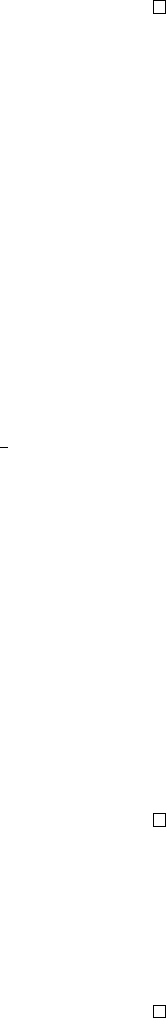4Quotient groups

IA Groups4.2 Quotient groups
Proposition.
Let
K C G
. Then the set of (left) cosets of
K
in
G
is a group
under the operation aK bK = (ab)K.
Proof.
First show that the operation is well-defined. If
aK
=
a
0
K
and
bK
=
b
0
K
,
we want to show that
aK bK
=
a
0
K b
0
K
. We know that
a
0
=
ak
1
and
b
0
=
bk
2
for some
k
1
, k
2
K
. Then
a
0
b
0
=
ak
1
bk
2
. We know that
b
1
k
1
b K
. Let
b
1
k
1
b
=
k
3
. Then
k
1
b
=
bk
3
. So
a
0
b
0
=
abk
3
k
2
(
ab
)
K
. So picking a different
representative of the coset gives the same product.
1. Closure: If aK, bK are cosets, then (ab)K is also a coset
2. Identity: The identity is eK = K (clear from definition)
3. Inverse: The inverse of aK is a
1
K (clear from definition)
4. Associativity: Follows from the associativity of G.
Definition
(Quotient group)
.
Given a group
G
and a normal subgroup
K
, the
quotient group or factor group of
G
by
K
, written as
G/K
, is the set of (left)
cosets of K in G under the operation aK bK = (ab)K.
Note that the set of left cosets also exists for non-normal subgroups (abnormal
subgroups?), but the group operation above is not well defined.
Example.
(i)
Take
G
=
Z
and
nZ
(which must be normal since
G
is abelian), the cosets
are
k
+
nZ
for 0
k < n
. The quotient group is
Z
n
. So we can write
Z/
(
nZ
) =
Z
n
. In fact these are the only quotient groups of
Z
since
nZ
are
the only subgroups.
Note that if G is abelian, G/K is also abelian.
(ii)
Take
K
=
hri C D
6
. We have two cosets
K
and
sK
. So
D
6
/K
has order 2
and is isomorphic to C
2
.
(iii)
Take
K
=
hr
2
i C D
8
. We know that
G/K
should have
8
2
= 4 elements. We
have
G/K
=
{K, rK
=
r
3
K, sK
=
sr
2
K, srK
=
sr
3
K}
. We see that all
elements (except K) has order 2, so G/K
=
C
2
× C
2
.
Note that quotient groups are not subgroups of
G
. They contain different
kinds of elements. For example,
Z/nZ
=
C
n
are finite, but all subgroups of
Z
infinite.
Example.
(Non-example) Consider
D
6
with
H
=
hsi
.
H
is not a normal
subgroup. We have
rH r
2
H
=
r
3
H
=
H
, but
rH
=
rsH
and
r
2
H
=
srH
(by
considering the individual elements). So we have
rsH srH
=
r
2
H 6
=
H
, and
the operation is not well-defined.
Lemma.
Given
K C G
, the quotient map
q
:
G G/K
with
g 7→ gK
is a
surjective group homomorphism.
Proof. q
(
ab
) = (
ab
)
K
=
aKbK
=
q
(
a
)
q
(
b
). So
q
is a group homomorphism.
Also for all aK G/K, q(a) = aK. So it is surjective.
Note that the kernel of the quotient map is
K
itself. So any normal subgroup
is a kernel of some homomorphism.
Proposition. The quotient of a cyclic group is cyclic.
Proof.
Let
G
=
C
n
with
H C
n
. We know that
H
is also cyclic. Say
C
n
=
hci
and
H
=
hc
k
i
=
C
`
, where
k`
=
n
. We have
C
n
/H
=
{H, cH, c
2
H, · · · c
k1
H}
=
hcHi
=
C
k
.